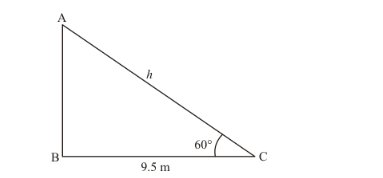# The angle of elevation of a ladder leaning against a wall is 60° and the foot

Question:

The angle of elevation of a ladder leaning against a wall is 60° and the foot of the ladder is 9.5 m away from the wall. Find the length of the ladder.

Solution:

Letbe the ladder of lengthm and C be the points, makes an angle of elevation 60° with the wall and foot of the ladder is 9.5 meter away from wall.

In a triangle ABC, given that BC = 9.5 m and angle C = 60°Now we have to find length of ladder.

So we use trigonometrically ratios.

In a triangle ABC,

$\Rightarrow \quad \cos C=\frac{B C}{A C}$

$\Rightarrow \cos 60^{\circ}=\frac{9.5}{h}$

$\Rightarrow \quad \frac{1}{2}=\frac{9.5}{h}$

$\Rightarrow \quad h=19$

Hence length of ladder is 19 meters.